# Using Python Visuals in Power BI

The addition of Python integration in Power BI is one of the greatest things that Microsoft could have done. This gives the user the ability to utilize amazing visual libraries such as Seaborn. Beyond visualization, you can also utilize some of the machine learning packages.

If you want to improve your data visualization skill, check out Use Power BI for Data Science.

Also, check out our blog on how to Do Machine Learning in Power BI.

The first thing you will need is the latest version of Power BI, this will have the Python integration in the preview features, you can enable this and then restart. Just go to your options and enable the feature.

## Use the Python Script Editor and Visual Tile

1. Click the Python visual within the Visualization panel. This will open the script editor window at the bottom of the page.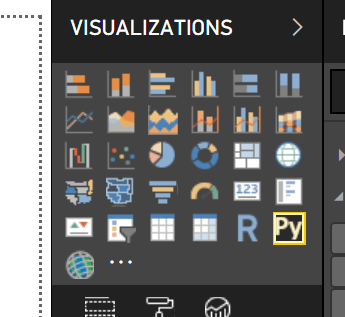When this icon is clicked, the script editor opens with a visual tile that is empty. I am using Google Analytics data to make my dataset.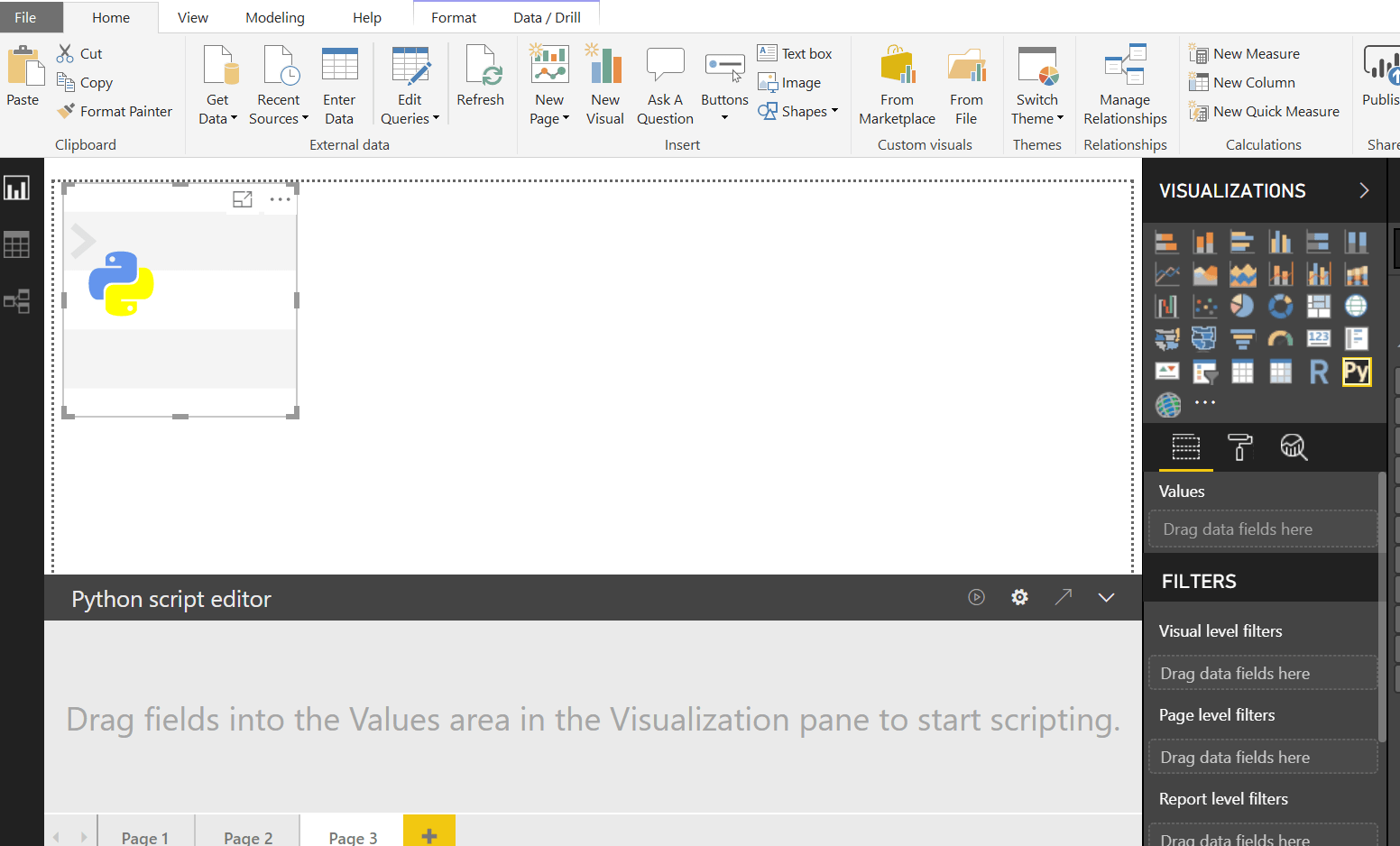2. You will need to add your values to build your dataset by selecting the fields which will fill your values section to create the data you will use in a visual.  Once you have the dataset built you will see it as a pandas data frame which can be thought of as a table.

3. Now you will need to import some libraries to ensure you can create the visuals. use the following script to import Matplotlib and Seaborn. We are using abbreviations of plt for and seaborn to make our code more efficient.

```import matploblib.pylot as plt
import seaborn as sns```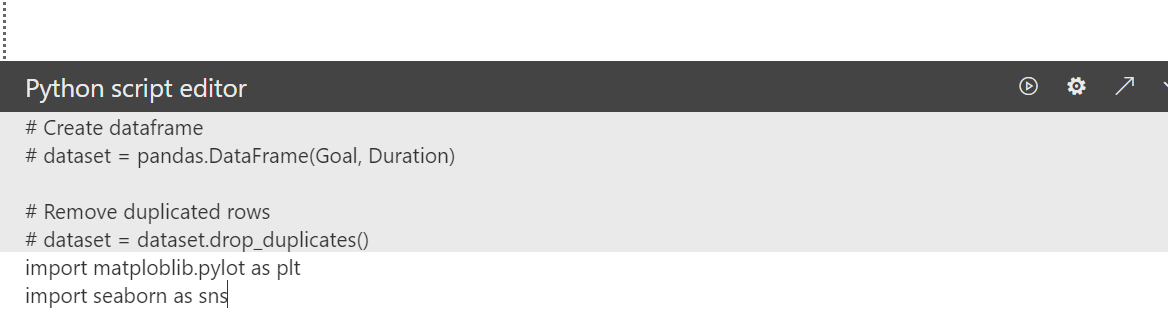### Violin Plot with Seaborn Libary

This imports the visuals library that will allow you to create some cool visuals. Now let’s build some plots aka visuals.  Since we have the first part of the code, let’s add to the code to get the visuals we want. The first visuals we will create is a violin plot.  We are going to use the values that we added in which creates our dataset.

Paste the Code:

```import matploblib.pylot as plt
import seaborn as sns
sns.violinplot(x='Device',y='Session Duration',data=dataset)
plt.show()```

Using this code will produce a violin plot which will show you where the concentration of session duration is on the y-axis by the device.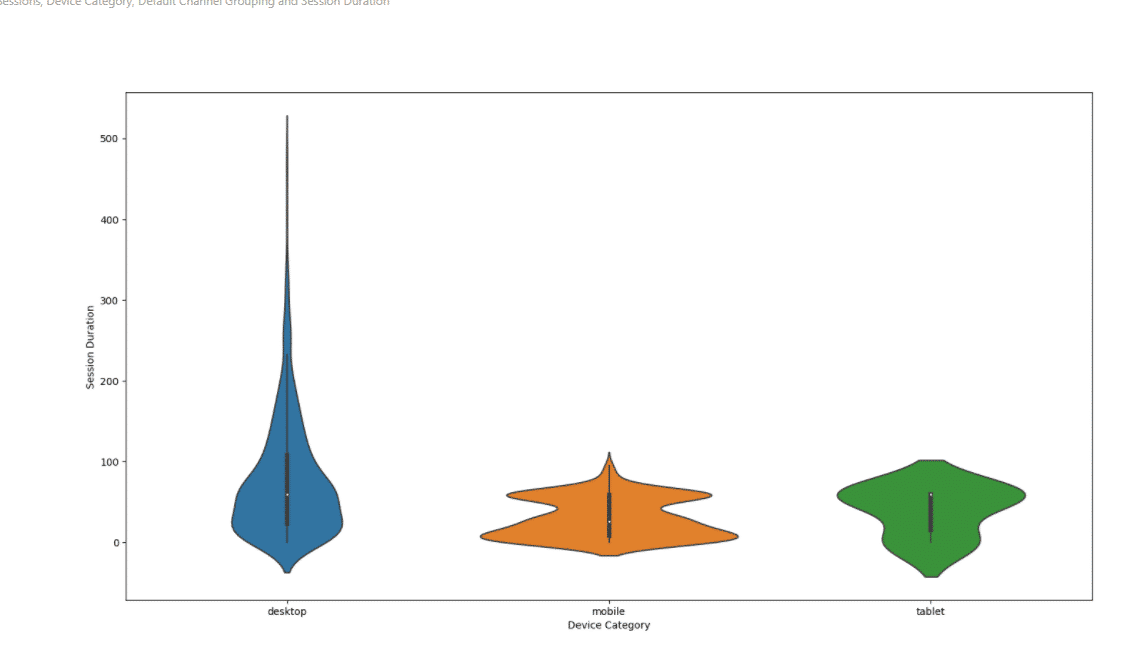We can see from the desktop has a higher amount of outliers with higher session durations than any other device. We can evaluate the gender by simply changing the x-axis to gender.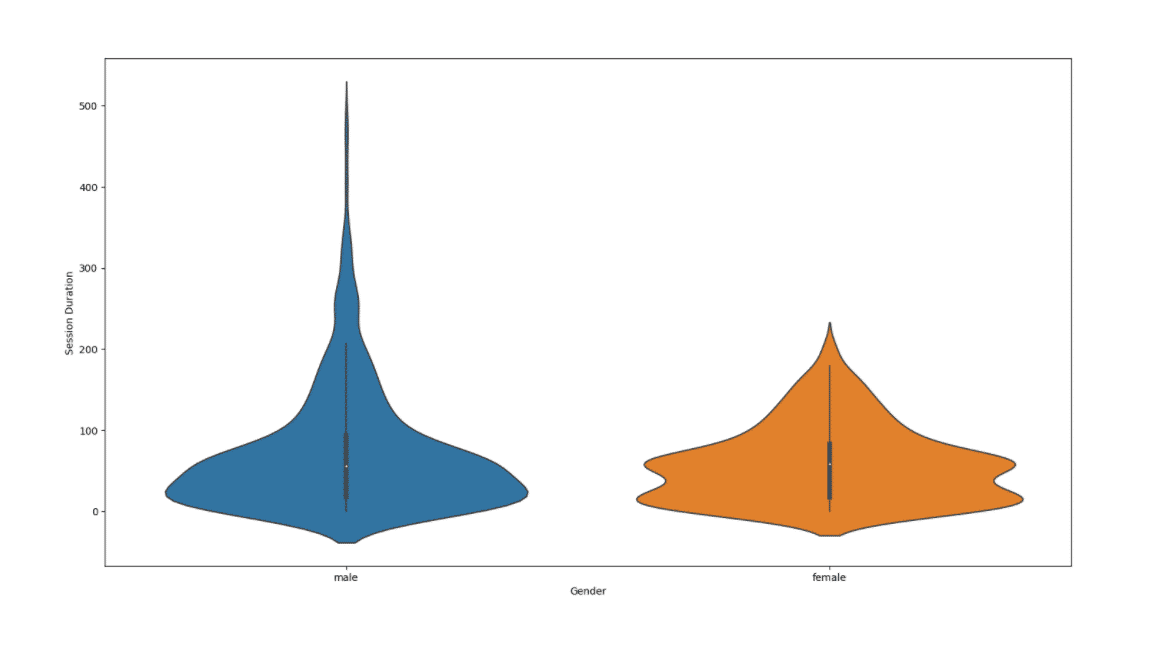### Create a Joint Plot

The next plot is going to joint plot. This works off the same backbone of code that you’ve already built using the violinplot.  The only difference is that you are going to exchange sns.violinplot for sns.jointplot.  However, a joint plot is a modified scatter plot so your X and Y variables will need numerical. For this example, I wanted to know if there was a correlation between session duration and goal completions.  you can see from my code below that I exchange that I used Session Duration as my x-axis and Goal Completions my y-axis

Paste the Code:

```import matplotlib.pyplot as plt
import seaborn as sns
sns.jointplot(x='Session Duration', y='Goal Completions', data=dataset)
plt.show()```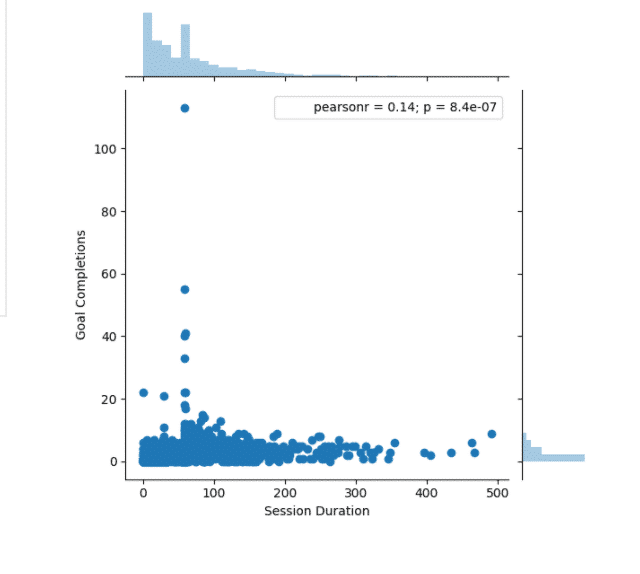This plot doesn’t award me a lot of insights into my goal completions. We can change the x variable into page views to see if we get a better correlation score which is listed in the top right.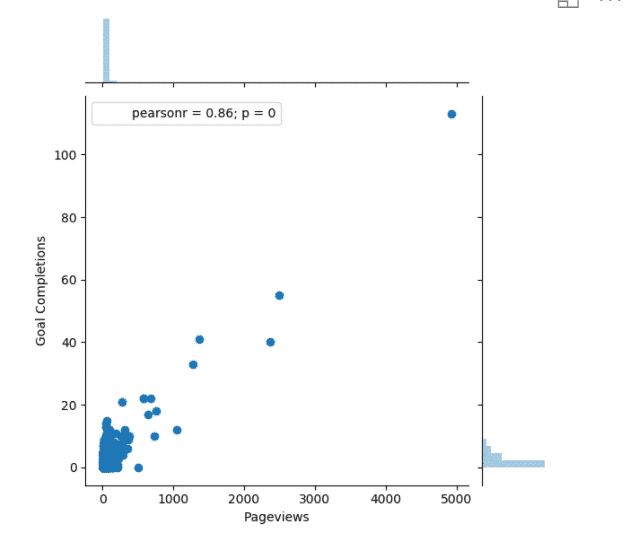### Create a Heatmap Correlation Plot

Now let’s create some correlation based on our dataset. This is a fun way to do some exploratory data analysis.  We can do this by using a heat map which is a very powerful visual. Since we are going to be evaluating the whole dataset, we don’t need to define the x and y-axis.  We can get a correlation score for every variable.

Paste the Code:

```import matplotlib.pyplot as plt
import seaborn as sns
sns.heatmap(dataset.corr(),cmap='coolwarm')
plt.show()```

The code takes in the whole dataset, I added a color gradient called ‘coolwarm’ however you can explore tons of color gradient. This was added with the cmap parameter. You can check out the Seaborn website to see other colors scales you can use.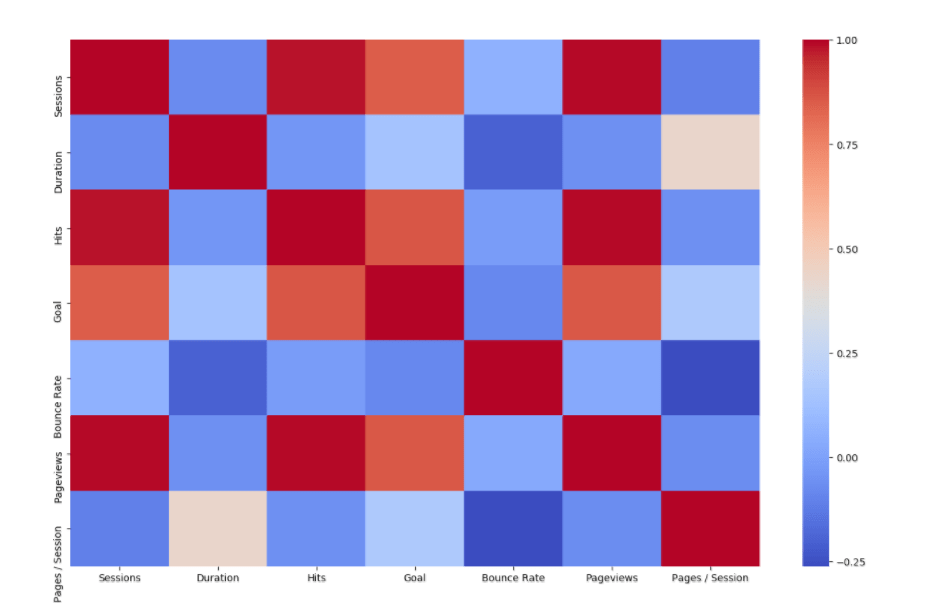We can further customize this visual by added no parameters such as labels and line width. This will make the heat map more actionable by displaying the correlations.

Paste the Code:

```import matplotlib.pyplot as plt
import seaborn as sns
sns.heatmap(dataset.corr(),cmap='coolwarm', annot = True, linewidth =0.7)
plt.show()```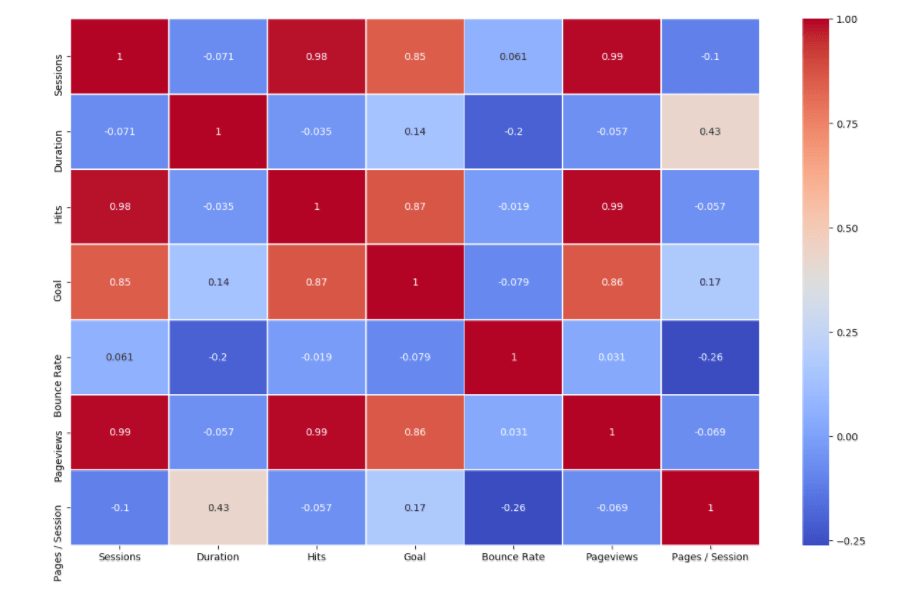### Create a Seaborn Pair Plot

The last plot we are going to create is the easiest. Its called a pair plot which is essentially an aggregation of all your dataset correlation similar in separate graphs. It gives you a quick and simple look at your correlations for deeper exploration.

Paste the Code:

```import matplotlib.pyplot as plt
import seaborn as sns
sns.pairplot(dataset)
plt.show()```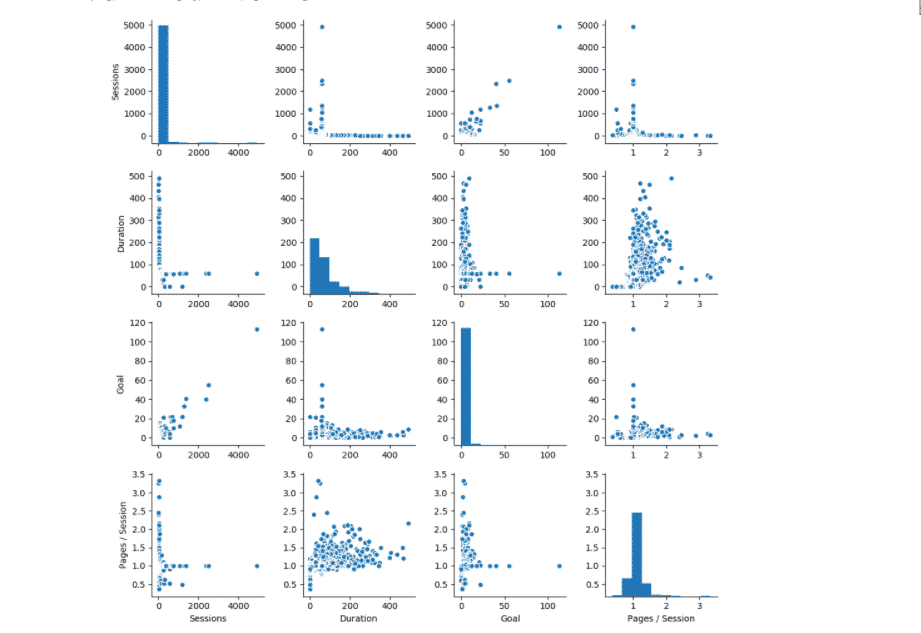Lastly, you can display these visuals as a report in any fashion that you like.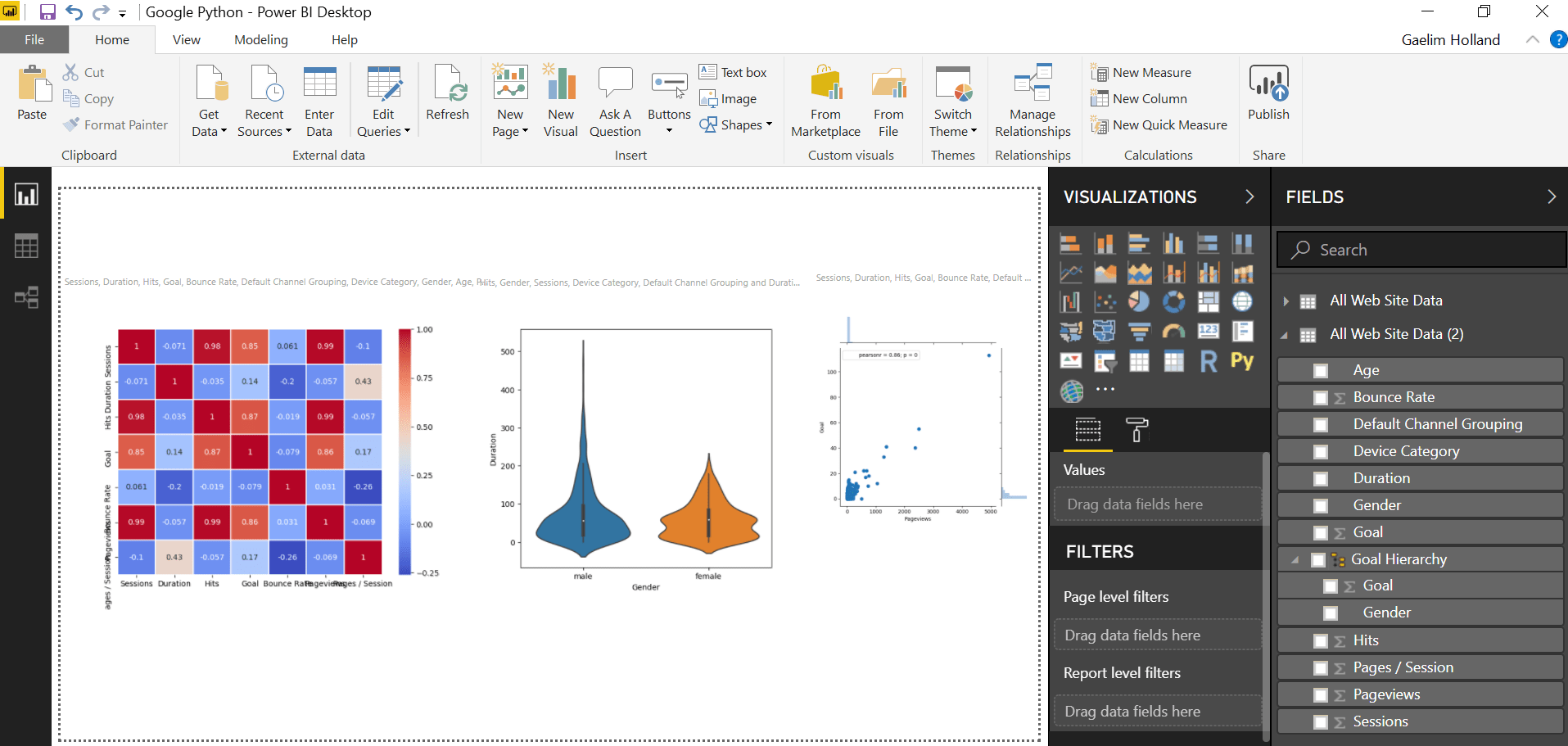Learn How to Load PDF Tables in Power BI